Home News About Me Ph. D. Thesis Abstract Table of Contents 1. Introduction 2. Theory  Fundamentals of the Multivariate Data Analysis 3. Theory  Quantification of the Refrigerants R22 and R134a: Part I 4. Experiments, Setups and Data Sets 5. Results  Kinetic Measurements 6. Results  Multivariate Calibrations 6.1. PLS Calibration 6.2. Box-Cox Transformation + PLS 6.3. INLR 6.4. QPLS 6.5. CART 6.6. Model Trees 6.7. MARS 6.8. Neural Networks 6.9. PCA-NN 6.10. Neural Networks and Pruning 6.11. Conclusions 7. Results  Genetic Algorithm Framework 8. Results  Growing Neural Network Framework 9. Results  All Data Sets 10. Results  Various Aspects of the Frameworks and Measurements 11. Summary and Outlook 12. References 13. Acknowledgements Publications Research Tutorials Downloads and Links Contact Search Site Map Print this Page## 6.3.   INLR

The Implicit Nonlinear PLS Regression (INLR) , is also called Nonlinear PLS in many publications. The INLR introduces nonlinearities into the regression model by adding squared terms (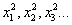) and optionally the cross-product terms (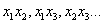) to the set of "original" independent variables (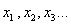) . For this study, only the squared terms were added as the addition of the cross-product terms would increase the number of independent variables to an unmanageable number of about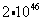. PLS models were built for the increased number of 100 independent variables with the optimal number of principal components selected by the minimum crossvalidation criterion.

The prediction of R22 by the optimal model with 16 principal components showed a relative error of 2.25% for the calibration data and 2.81% for the validation data. For R134a the optimal model with 17 principal components predicted the calibration data with a rel. RMSE of 3.47% and the validation data with a rel. RMSE of 4.02%. The addition of the squared variables can be also seen as a polynomial approach, which might explain why a rather many principal components are needed. This high number of principal components increases the relative gap between the error for the calibration data and the validation data compared with the Box-Cox Transformation and PLS due to the increased number of parameters (see also section 2.8.1). Yet, the INLR compen­sates the nonlinearities better than these two methods, as only for R22 the Wald-Wolfowitz Runs test and the Durbin-Watson Statistics are significant.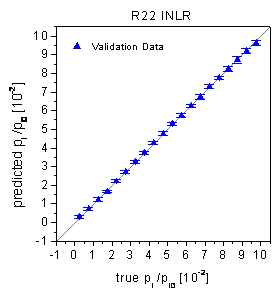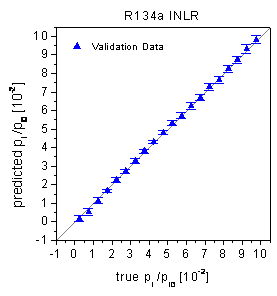figure 35:  True-predicted plots of the INLR for the validation data.

 Page 92 © Frank Dieterle, 03.03.2019